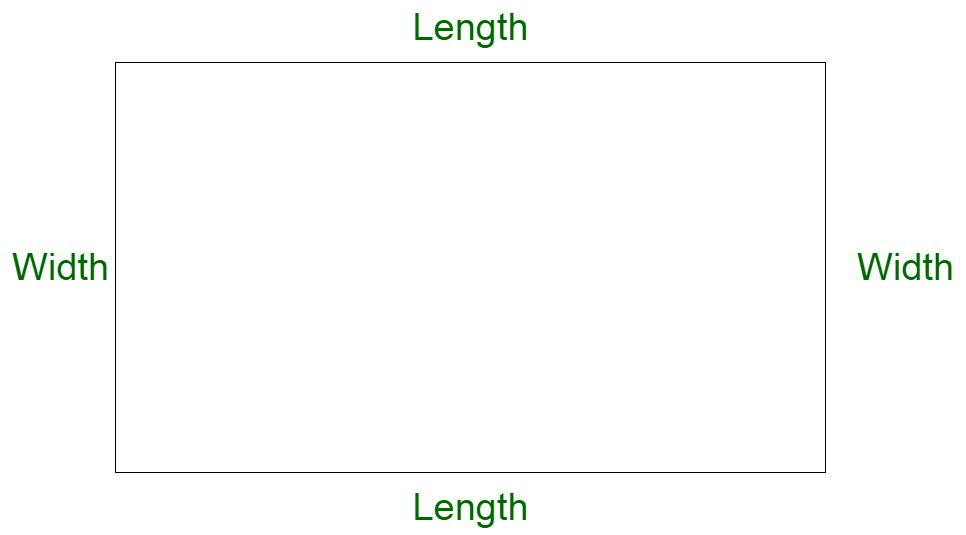GeeksforGeeks App
Open AppBrowser
Continue

# Find the area of the rectangle if the breadth is 19 cm and length is 3 times longer than its breadth.

Rectangle is a closed two-dimensional figure composed of four sides and four vertices. All angles of the rectangle are 900. A rectangle with all sides equal is equivalent to a square. A rectangle is composed of two pairs of parallel sides, length and breadth respectively.

Properties of rectangle

• It has four sides and four angles.
• All the angles are right angles.
• Opposite sides are parallel and equal in length.

Area of Rectangle

A rectangle is composed of equal pairs which are parallel in nature and equal in length. The area of a rectangle is the space enclosed within its boundaries. The area of the rectangle is the product of the length and breadth of the rectangle.

Area of rectangle = Length × Breadth

Let us assume A to be the area of the rectangle and l and b to be the length and breadth of the rectangle respectively.

A = l × b### Find the area of the rectangle if the breadth is 19 cm and the length is 3 times longer than its breadth.

Solution:

Let us assume l to be the length of the rectangle and b to be the breadth of the rectangle.

Given values,

And,

Length is three times longer than its breadth.

Length = 3 × 19 cm

Length, l = 57 cm

Now,

A = l × b

A = 19 × 57 cm2

A = 1083 cm2

### Sample Questions

Question 1: If the area becomes quadruple, keeping the length the same, how does the breadth change?

Solution:

Area’ = 4 × Area

Now,

Given , length is constant, length’ = length

Therefore,

Question 2: The length and breadth are in the ratio of 2:3. The area is 12 cm. Calculate the length and breadth.

Solution :

Let the item be x. Now length = 2x and breadth = 3x respectively.

We know,

2x × 3x = 12

6x2 = 12

x2 = 2

x = √2

Question 3: The breadth of a rectangle is 3 cm. Its length is 2/3 times longer than its breadth. Find the area of the rectangle.

Solution:

Area of rectangle = Length × Breadth

Now, given,

= 2/3 × 3 cm

= 2 cm

= 2 × 3 cm2

= 6 cm2

Question 4: Find the area of a rectangle when its length is 4 times its breadth. The breadth of the rectangle is 10 m?

Solution:

Here we have to find the area of the rectangle

As per the question

Length of the rectangle is 4 times the breadth

Thus,

Length of rectangle = 4 × breadth

Length of rectangle = 4 × 10

Length of rectangle = 40 m

As we know that

Area of rectangle = Length × Breadth

Area of rectangle = 40 × 10

Area of rectangle = 400 m2

Therefore,

Area of rectangle is 400 m2

Question 5: The breadth of a rectangle is 4 cm. Its length is two units longer than its breadth. Find the area of the rectangle.

Solution:

Area of rectangle = Length × Breadth

Now, given,

Length = Breadth + 2 cm

= 4 cm × 2 cm

= 6 cm